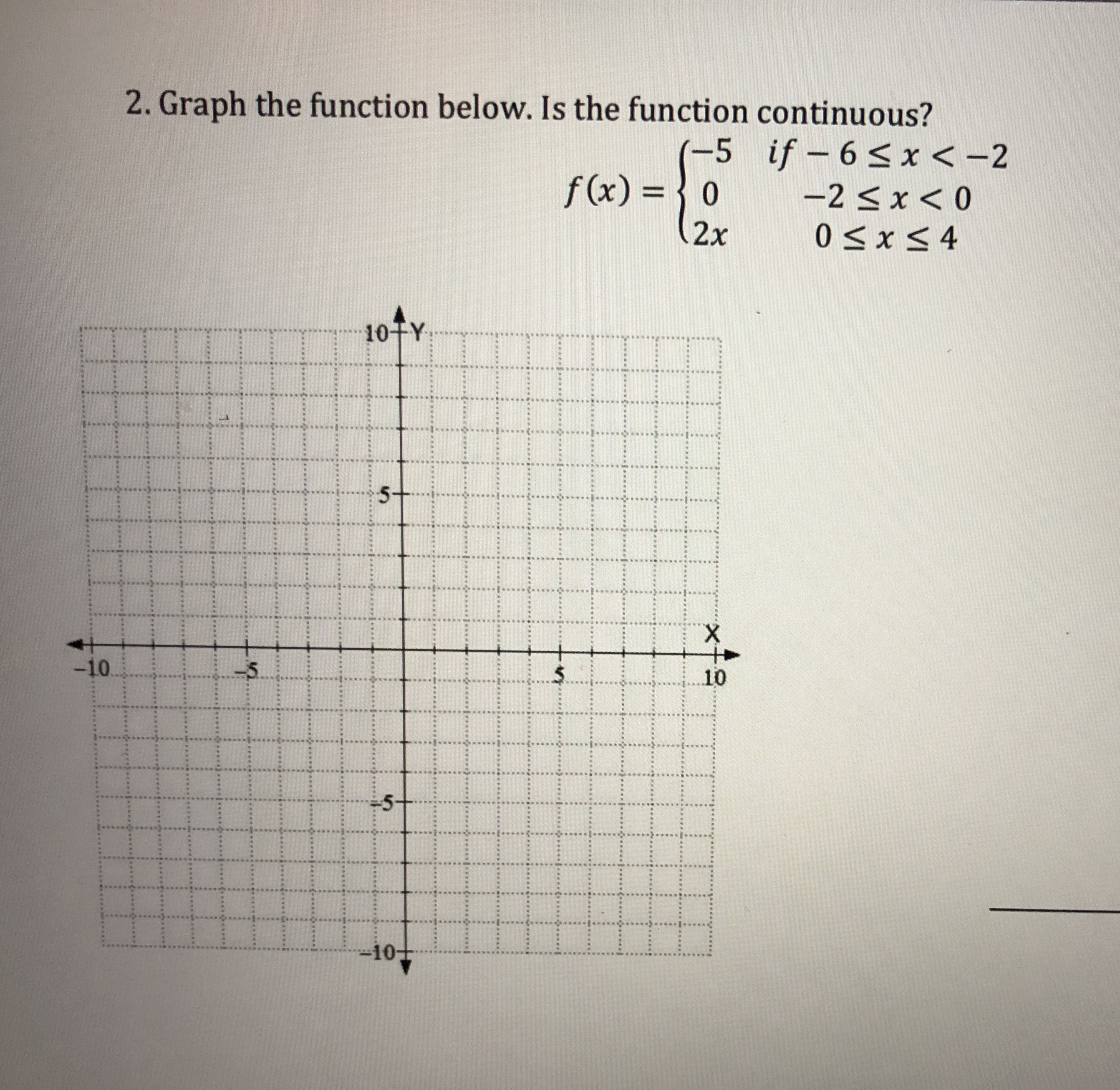# Graph the function below. Is the function continuous?f (x)o2x10TY51010

Question
2 viewshelp_outlineImage TranscriptioncloseGraph the function below. Is the function continuous? f (x)o 2x 10TY 5 10 10 fullscreen
check_circle

Step 1

To represent the given function on the coordinate plane.

Step 2

Note that the function is defined differently in the three different intervals specified. Therefore , the graph of the function will consist of three different pieces .

Step 3

First draw the graph for the interval x in [-6,-2); this is the constant function (-5) . Note that t...

### Want to see the full answer?

See Solution

#### Want to see this answer and more?

Solutions are written by subject experts who are available 24/7. Questions are typically answered within 1 hour.*

See Solution
*Response times may vary by subject and question.
Tagged in
MathAlgebra

### Equations and In-equations